Tools, Technologies and Training for Healthcare Laboratories

# Z-5: Sum of Squares, Variance, and the Standard Error of the Mean

When you compare monthly QC data or perform initial method validation experiments, you do a lot of mean comparison. Dr. Madelon F. Zady, Ph.D., talks about the means of means and other important statistical calculations.

### Mean or average

The previous lesson described the calculation of the mean, SD, and CV and illustrated how these statistics can be used to describe the distribution of measurements expected from a laboratory method. A common application of these statistics is the calculation of control limits to establish the range of values expected when the performance of the laboratory method is stable. Changes in the method performance may cause the mean to shift the range of expected values, or cause the SD to expand the range of expected values. In either case, individual control values should exceed the calculated control limits (expected range of values) and signal that something is wrong with the method.

The calculation of a mean is linked to the central location or correctness of a laboratory test or method (accuracy, inaccuracy, bias, systematic error, trueness) and the calculation of an SD is often related to the dispersion or distribution of results (precision, imprecision, random error, uncertainty). In estimating the central location of a group of test results, one could attempt to measure the entire population or to estimate the population parameters from a smaller sample. The values calculated from the entire population are called parameters (mu for the mean, sigma for the standard deviation), whereas the values calculated from a smaller sample are called statistics (Xbar for the mean, SD for the standard deviation).

### A simulated experiment

Consider the situation where there are 2000 patients available and you want to estimate the mean for that population. Blood specimens could be drawn from all 2000 patients and analyzed for glucose, for example. This would be a lot of work, but the whole population could be tested and the true mean calculated, which would then be represented by the Greek symbol mu (µ). Assume that the mean (µ) for the whole population is 100 mg/dl. How close would you be if you only analyzed 100 specimens?

This situation can be demonstrated or simulated by recording the 2000 values on separate slips of paper and placing them in a large container. You then draw out a sample of 100 slips of paper, calculate the mean for this sample of 100, record that mean on a piece of paper, and place it in a second smaller container. The 100 slips of paper are then put back into the large container with the other 1900 (a process called with sampling with replacement) and the container shuffled and mixed. You then draw another sample of 100 slips from the large container, calculate the mean, record the mean on paper, place that slip of paper in the small container, return the 100 slips of paper to the large container, and shuffle and mix. If you repeat this process ten more times, the small container now has 12 possible estimates of the "sample of 100" means from the population of 2000.

### Calculation of the mean of a sample (and related statistical terminology)

We will begin by calculating the mean and standard deviation for a single sample of 100 patients. The mean and standard deviation are calculated as in the previous lesson, but we will expand the statistical terminology in this discussion. The table below shows the first 9 of these values, where X is an individual value or score, Xbar is the mean, and X minus Xbar is called the deviation score or delta (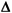).

 Calculation of the mean of a "sample of 100" Column A Value or Score(X) Column B Deviation Score () (X-Xbar) Column CDeviation Score² (²) (X-Xbar)² 100 100-94.3 = 5.7 (5.7)² = 32.49 100 100-94.3 = 5.7 (5.7)² = 32.49 102 102-94.3 = 7.7 (7.7)² = 59.29 98 98-94.3 = 3.7 (3.7)² = 13.69 77 77-94.3 = -17.3 (-17.3)² = 299.29 99 99-94.3 = 4.7 (4.7)² = 22.09 70 70-94.3 = -24.3 (-24.3)² = 590.49 105 105-94.3 = 10.7 (10.7)² = 114.49 98 98-94.3 = 3.7 (3.7)² = 3.69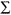Xor(X-Xbar)² or(X-Xbar)² "first moment" Sum of Squares (SS)
• Scores. Column A provides the individual values or scores are used to calculate the mean.
• Mean. The sum of the scores is divided by the number of values (N=100 for this example) to estimate the mean, i.e.,X/N = mean.
• Deviation scores. Column B represents the deviation scores, (X-Xbar), which show how much each value differs from the mean. In lesson four we called these the difference scores. They are also sometimes called errors (as will be seen later in this lesson).
• First moment. The sum of the deviation scores is always zero. This zero is an important check on calculations and is called the first moment. (The moments are used in the Pearson Product Moment Correlation calculation that is often used with method comparison data.)
• Sum of squares. The third column represents the squared deviation scores, (X-Xbar)², as it was called in Lesson 4. The sum of the squared deviations,(X-Xbar)², is also called the sum of squares or more simply SS. SS represents the sum of squared differences from the mean and is an extremely important term in statistics.
• Variance. The sum of squares gives rise to variance. The first use of the term SS is to determine the variance. Variance for this sample is calculated by taking the sum of squared differences from the mean and dividing by N-1: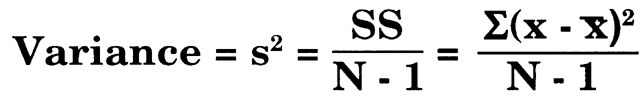• Standard deviation. The variance gives rise to standard deviation. The second use of the SS is to determine the standard deviation. Laboratorians tend to calculate the SD from a memorized formula, without making much note of the terms.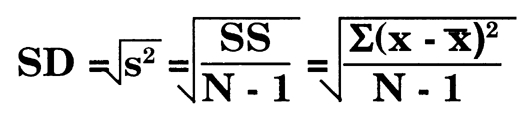It's important to recognize again that it is the sum of squares that leads to variance which in turn leads to standard deviation. This is an important general concept or theme that will be used again and again in statistics. The variance of a quantity is related to the average sum of squares, which in turn represents sum of the squared deviations or differences from the mean.

### Calculation of the mean of the means of samples (the standard error of the mean)

Now let's consider the values for the twelve means in the small container. Let's calculate the mean for these twelve "mean of 100" samples, treating them mathematically much the same as the prior example that illustrated the calculation of an individual mean of 100 patient values.

 Calculation of the mean of the twelve means from "samples of 100" Column AXbarValues Column BXbar-µ Deviations Column C(Xbar-µ)²Deviations squared 100 100-100 = 0 0 99 99-100 = -1 (-1)² = 1 98 98-100 = -2 (-2)² = 4 106 106-100 = 6 (6)² = 36 97 97-100 = -3 (-3)² = 9 95 95-100 = -5 (-5)² = 25 99 99-100 = -1 (-1)² = 1 101 101-100 = 1 (1)² = 1 97 97-100 = -3 (-3)² = + 9 96 96-100 = -4 (-4)² = 16 100 100-100 = 0 0 100 100-100 = 0 0Xbar=1188= 0 SS = 102
• Mean of means. Remember that Column A represents the means of the 12 samples of 100 which were drawn from the large container. The mean of the 12 "samples of 100" is 1188/12 or 99.0 mg/dl.
• Deviations or errors. Column B shows the deviations that are calculated between the observed mean and the true mean (µ = 100 mg/dL) that was calculated from the values of all 2000 specimens.
• Sum of squares. Column C shows the squared deviations which give a SS of 102.
• Variance of the means. Following the prior pattern, the variance can be calculated from the SS and then the standard deviation from the variance. The variance would be 102/12, which is 8.5 (Note that N is used here rather than N-1 because the true mean is known). Mathematically, it is SS over N.
• Standard deviation of the means, or standard error of the mean. Continuing the pattern, the square root is extracted from the variance of 8.5 to yield a standard deviation of 2.9 mg/dL. This standard deviation describes the variation expected for mean values rather than individual values, therefore, it is usually called the standard error of the mean, the sampling error of the mean, or more simply the standard error (sometimes abbreviated SE). Mathematically it is the square root of SS over N; statisticians take a short cut and call it s over the square root of N.
•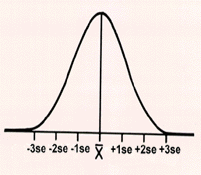Sampling distribution of the means. If from the prior example of 2000 patient results, all possible samples of 100 were drawn and all their means were calculated, we would be able to plot these values to produce a distribution that would give a normal curve. The sampling distribution shown here consists of means, not samples, therefore it is called the sampling distribution of means.

### Why are the standard error and the sampling distribution of the mean important?

Important statistical properties. Conclusions about the performance of a test or method are often based on the calculation of means and the assumed normality of the sampling distribution of means. If enough experiments could be performed and the means of all possible samples could be calculated and plotted in a frequency polygon, the graph would show a normal distribution. However, in most applications, the sampling distribution can not be physically generated (too much work, time, effort, cost), so instead it is derived theoretically. Fortunately, the derived theoretical distribution will have important common properties associated with the sampling distribution.

• The mean of the sampling distribution is always the same as the mean of the population from which the samples were drawn.
• The standard error of the mean can be estimated by the square root of SS over N or s over the square root of N or even SD/(N)1/2. Therefore, the sampling distribution can be calculated when the SD is well established and N is known.
• The distribution will be normal if the sample size used to calculate the mean is relatively large, regardless whether the population distribution itself is normal. This is known as the central limit theorem. It is fundamental to the use and application of parametric statistics because it assures that - if mean values are used - inferences can be made on the basis of a gaussian or normal distribution.
• These properties also apply for sampling distributions of statistics other than means, for example, variance and the slopes in regression.

In short, sampling distributions and their theorems help to assure that we are working with normal distributions and that we can use all the familiar "gates."

Important laboratory applications. These properties are important in common applications of statistics in the laboratory. Consider the problems encountered when a new test, method, or instrument is being implemented. The laboratory must make sure that the new one performs as well as the old one. Statistical procedures should be employed to compare the performance of the two.

• Initial method validation experiments that check for systematic errors typically include recovery, interference, and comparison of methods experiments. The data from all three of these experiments may be assessed by calculation of means and comparison of the means between methods. The questions of acceptable performance often depend on determining whether an observed difference is greater than that expected by chance. The observed difference is usually the difference between the mean values by the two methods. The expected difference can be described by the sampling distribution of the mean.
• Quality control statistics are compared from month to month to assess whether there is any long-term change in method performance. The mean for a control material for the most recent month is compared with the mean observed the previous month or the cumulative mean of previous months. The change that would be important or significant depends on the standard error of the mean and the sampling distribution of the means.
• Comparisons between laboratories are possible when common control materials are analyzed by a group of laboratories - a program often called peer comparison. The difference between the mean of an individual laboratory and the mean of the group of laboratories provides an estimate of systematic error or inaccuracy. The significance of an individual difference can be assessed by comparing the individual value to the distribution of means observed for the group of laboratories.

### Self-assessment questions

1. What does SS represent? Describe it in words. Express it mathematically.
2. Why is the concept sum of squares (SS) important?
3. Show how the variance is calculated from the SS.
4. Show how the SD is calculated from the variance and SS.
5. What's the difference between the standard deviation and the standard error of the mean?
6. Given a method whose SD is 4.0 mg/dL and 4 replicate measurements are made to estimate a test result of 100 mg/dL, calculate the standard error of the mean to determine the uncertainty of the test result.